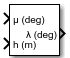# Geodetic to Geocentric Latitude

Convert geodetic latitude to geocentric latitude

• Library:
• Aerospace Blockset / Utilities / Axes Transformations

•## Description

The Geodetic to Geocentric Latitude block converts a geodetic latitude (μ) into geocentric latitude (λ). For more information on the geocentric latitude, see Algorithms.

## Limitations

This block implementation generates a geocentric latitude that lies between ±90 degrees.

## Ports

### Input

expand all

Geodetic latitude, specified as a scalar, in degrees. Latitude values can be any value. However, values of +90 and -90 may return unexpected values because of singularity at the poles.

Data Types: `double`

Mean sea-level altitude (MSL), specified as a scalar.

Data Types: `double`

### Output

expand all

Contains the geocentric latitude, specified as a scalar, in degrees.

Data Types: `double`

Radius from center of the planet to the center of gravity, returned as a scalar.

#### Dependencies

To enable this port, select Output radius.

Data Types: `double`

## Parameters

expand all

Parameter and output units:

Units

Radius from CG to Center of Planet

`Metric (MKS)`

Meters

Meters

`English`

Feet

Feet

#### Programmatic Use

 Block Parameter: `units` Type: character vector Values: ```'Metric (MKS)'``` | `'English'` Default: ```'Metric (MKS)'```

Planet model to use, `Custom` or `Earth (WGS84)`.

#### Dependencies

Selecting the `Custom` option enables these parameters:

• Flattening

#### Programmatic Use

 Block Parameter: `ptype` Type: character vector Values: ```'Earth (WGS84)'``` | `'Custom'` Default: ```'Earth (WGS84)'```

Flattening of the planet, specified as a double scalar.

#### Dependencies

This parameter is enabled when Planet model is set to `Custom`.

#### Programmatic Use

 Block Parameter: `F` Type: character vector Values: double scalar Default: `1/298.257223563`

Radius of the planet at its equator, in the same units as the Units parameter.

#### Dependencies

This parameter is enabled when Planet model is set to `Custom`.

#### Programmatic Use

 Block Parameter: `R` Type: character vector Values: double scalar Default: `6378137`

Select this check box to output the scalar distance radius from the equatorial radius to the center of the planet.

#### Dependencies

Select this check box to enable the r port.

#### Programmatic Use

 Block Parameter: `outputRadius` Type: character vector Values: `off` | `on` Default: `'off'`

## Algorithms

The Geodetic to Geocentric Latitude block converts a geodetic latitude (μ) into geocentric latitude (λ), where:

• λ — Geocentric latitude

• μ — Geodetic latitude

• h — Height from the surface of the planet

• f — Flattening

• a — Equatorial radius of the plant (semi-major axis)

Given the geodetic latitude (μ) and the height from the surface of the planet (h), this block first calculates the geometric properties of the planet.

`$\begin{array}{l}{e}^{2}=\frac{f}{\left(2-f\right)}\\ N=\frac{a}{\sqrt{1-{e}^{2}\mathrm{sin}{\left(\mu \right)}^{2}}\right)}.\end{array}$`

It then calculates the geocentric latitude from the point's distance from the polar axis (ρ) and distance from the equatorial axis (z).

`$\begin{array}{l}\rho =\left(N+h\right)\mathrm{sin}\left(\mu \right)\\ z=\left(N\left(1-{e}^{2}\right)+h\right)\mathrm{sin}\left(\mu \right)\\ \lambda ={\mathrm{tan}}^{-1}\left(\frac{z}{\rho }\right).\end{array}$`

 Stevens, B. L., and F. L. Lewis. Aircraft Control and Simulation, Hoboken, NJ: John Wiley & Sons, 1992.

## Support평가판 신청